# Distributional and frequency effects in word embeddings: Similarity stratification¶

## Overview¶

This is one of a series of posts. In this post I look at what I call stratification of similarities and ranks, as well the "reciprocity" of ranks (how close the ranks are when we swap the reference and the comparison). What we observe is that in many cases the similarity (or rank) of two words is related to their relative frequencies.

While  noticed a related phenomenon, which I'll turn to in the next post, I believe this is the first time that stratification of similarities and ranks has been noticed.

### Results and contributions¶

• new Stratification of similarities
• for sgns and ft, frequency is inversely related to similarity
• for glove and ppmi frequency is directly related to similarity
• new Stratification of rank
• for all models, the frequency of the reference term is somewhat directly correlated to rank
• for sgns and ft, the frequency of the comparison term is inversely related to to rank
• for glove and ppmi, the frequency of comparison term is inversely related to to rank
• for glove and ppmi, there is a smaller difference between reference and comparison terms than with sgns and ft
• new Stratification of reciprocity
• for sgns and ft, words with similar relative frequencies are more reciprocal than words with different relative frequencies
• for glove, there is general reciprocity, except when one word is very low frequency
• ppmi patterns more with sgns and ft than with glove, contrary to expectations based on rank
• new Behavior with respect to corpora for rank and reciprocity
• ft, glove, and ppmi behave similarily for both corpora
• sgns behaves differently the corpora, patterning with ft for vfair, but with glove and ft for heartd

Download as Jupyter notebook

Download supplemental Python code

Show Code

In :
#imports
from dfewe import *

#for tables in Jupyter
from IPython.display import HTML, display
import tabulate

In :
# some utilities
def show_title(t):
display(HTML('<b>%s</b>' % t))

def show_table(data,headers,title):
show_title(title)
display(HTML(tabulate.tabulate(data,tablefmt='html', headers=headers)))

In :
#set up standard corpora + vectors
vfair_all = Setup.make_standard_sampler_and_vecs('vfair',5,100,1) #window=5, dims=100, min_count=1
heartd_all = Setup.make_standard_sampler_and_vecs('heartd',5,100,1) #window=5, dims=100, min_count=1

what = [['Vanity Fair (vfair)'],['Heart of Darkness (heartd)']]
for i,c in enumerate([vfair_all,heartd_all]):
sampler = c['sampler']
what[i].extend([sum(sampler.counts.values()), len(sampler.counts)])

show_table(what, headers=['Corpus','Tokens','Types'], title="Corpora sizes")

Corpora sizes
Corpus Tokens Types
Vanity Fair (vfair) 310722 15803
Heart of Darkness (heartd) 38897 5420

## Stratification of similarities¶

We saw in the previous post that removing low frequency words lowered the mean of the similarities. That means that low frequency words contribute higher than average similarities. We can look for a broader relation between word frequency and similarities by looking at the mean similarity of words by their relative frequencies. Below we have a heatmap showing the mean similarities by percentile bands for sgns vectors from Vanity Fair (vfair). The darker the square, the higher the mean similarity for words in the corresponding percentil bands. For example, pairs of words both in the 0-5 percentile band have a mean similarity of over 0.9, while pairs words in the 0-5 percentile band and the 85-90 percentile band have a mean similarity of about 0.55.

In :
samples = 1000
step = 5
Plotting.show_range_comparison(vfair_all['sampler'],vfair_all['sgns'],'vfair, sgns',samples=samples,step=step, full_range=False)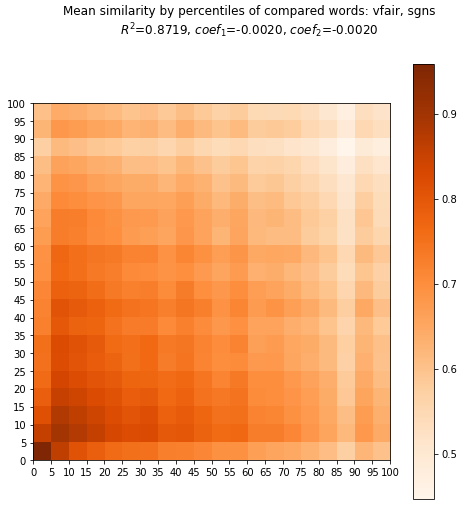While there is a particular anomaly in the 85-90 percentile band, and a few other small anomalies, what we see is that overall the colors get lighter from left to right and from bottom to top. That means that overall the pattern is that comparing word w to a lower frequency word will give a higher similarity than comparing w to a higher frequency word. In other words, frequency is inversely related to similarity for these vectors, which we can also see by the negative coefficients of the linear regression.

Let's compare all 4 methods for vfair.

In :
samples = 1000
step = 5
Plotting.compare_range_comparisons(vfair_all,'vfair',samples=samples, step=step, full_range=False)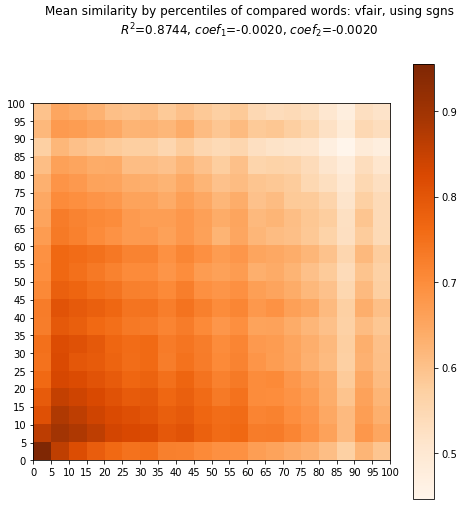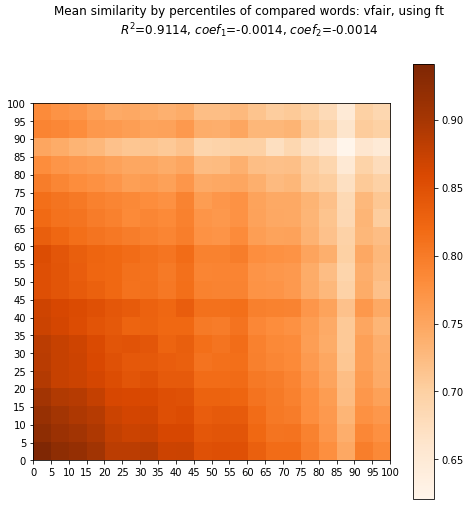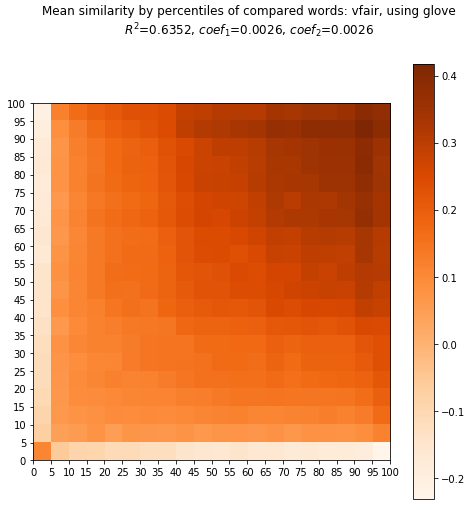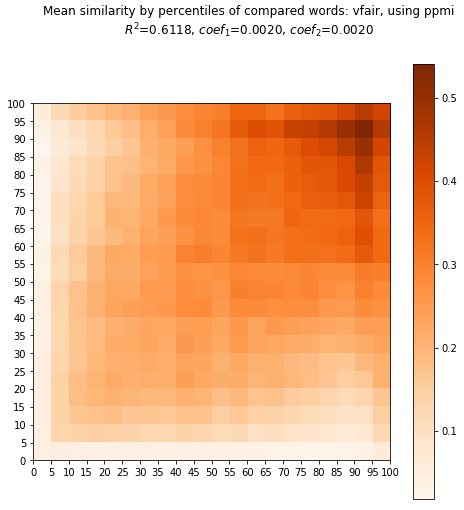The most striking thing to notice in comparing the four methods is that sgns and ft have similar patterns, but glove and ppmi have the opposite pattern. So while for sgns and ft frequency is inversely related to similarity, for glove and ppmi frequency is directly related to similarity (with positive coefficients in the regression). Note that this difference cannot be attributed simply to the differences in overall means that we saw in the previous post: it is logically/mathematically possible to have the inverse relation with a low average mean and a direct relation with a high average mean.

As in our first example with sgns, there are various anomalies. In addition, glove and ppmi have a strong anomaly in the 90-95 percentile band, whereas for sgns and ppmi the (corresponding?) anomaly is in the 85-90 percentile band.

Now let's do the same comparison, but using Heart of Darkness (heartd).

In :
samples = 1000
step = 5
Plotting.compare_range_comparisons(heartd_all,'heartd',samples=samples, step=step, full_range=False)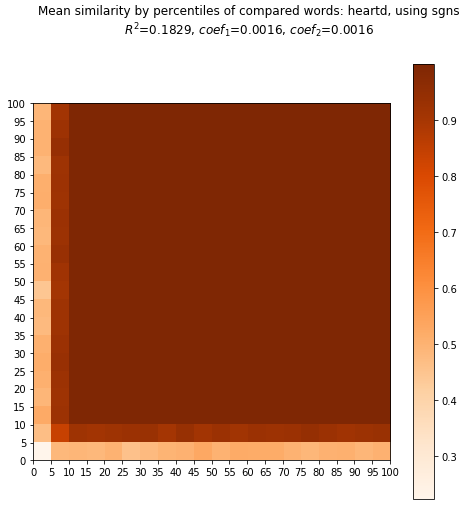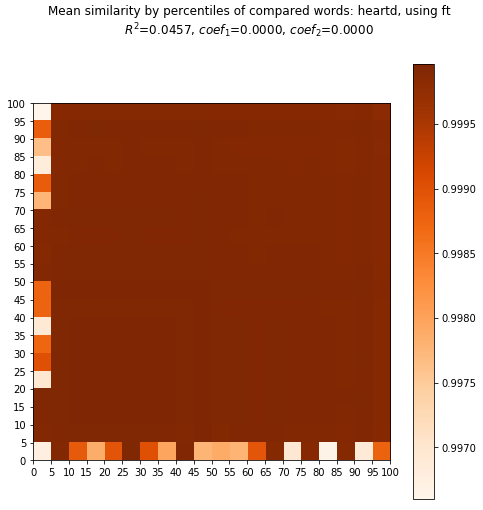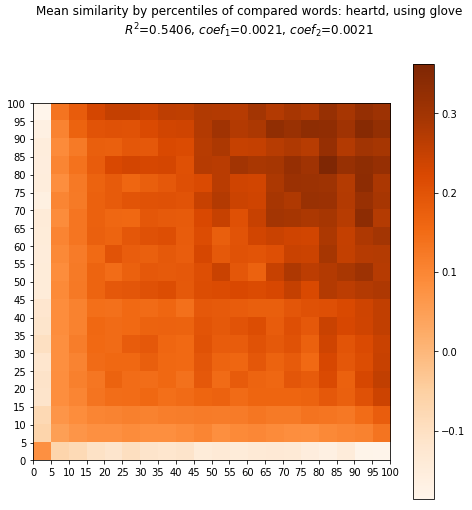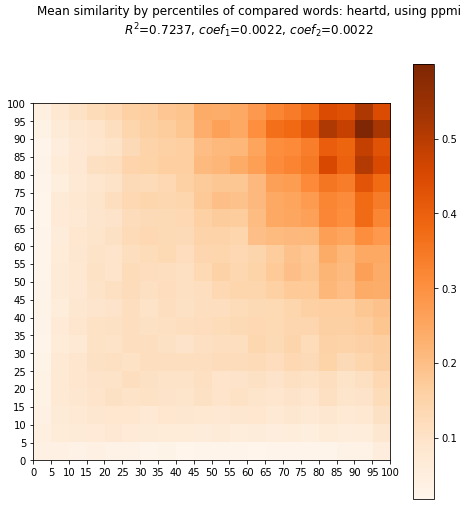What we see is that sgns and ft do not discriminate well for Heart of Darkness, but this is not surprising given the overall distributions that we saw in the previous post. We also see that glove and ppmi have similar (though attenuated) patterns for both texts. This suggests that the relations between frequency and similarity are properities of the methods rather than of the texts. Of course, this would need to be verified by looking at more corpora.

## Stratification of ranks¶

Often we are interested in the rank of a word with respect to another word rather than their similarity. An important thing about rank is that is not, in general, symmetric, unlike similarity.

In :
vecs = vfair_all['sgns']
(word1, word2) = ('lady','woman')
print('Similarity of "%s" and "%s": %0.4f' % (word1,word2,vecs.similarity(word1,word2)))
print('Rank of "%s" with respect to "%s": %d' % (word1,word2,vecs.rank(word1,word2)))
print('Rank of "%s" with respect to "%s": %d' % (word2,word1,vecs.rank(word2,word1)))

Similarity of "lady" and "woman": 0.6593
Rank of "lady" with respect to "woman": 409
Rank of "woman" with respect to "lady": 8072


Again, let's start with a look at the sgns vectors for vfair. In these heatmaps, the "reference" word is the "with respect to" word, and the comparison is the other word. So in the first example above, the rank of lady with respect to woman, woman is the reference and lady is the comparison. Note too that I'm using relative rank in order to be able to compare vocabularies of different sizes (e.g. vfair vs heartd).

In :
sampler = vfair_all['sampler']
vecs = vfair_all['sgns']
samples = 100 #fewer, because this is slow
step = 5
Plotting.show_relrank_comparison(sampler,vecs,'vfair',samples=samples,step=step, full_range=False)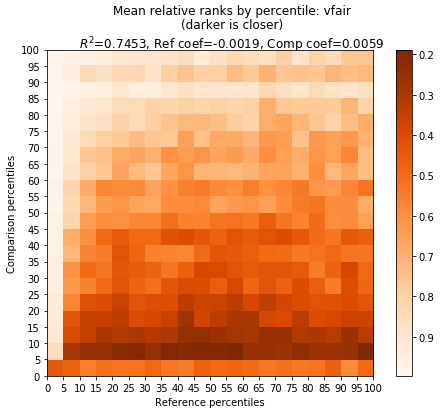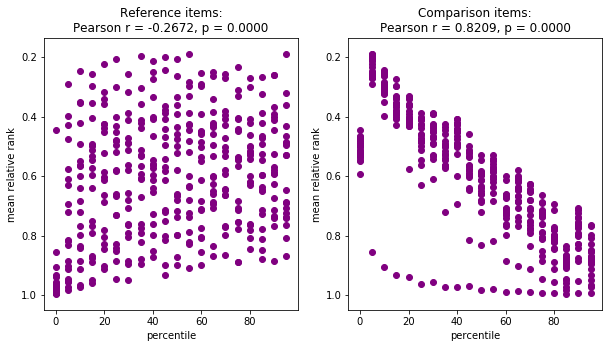We can see in the heatmap above that in contrast to the similarities, the mean relative ranks is not symmetrical. However, there are patterns concerning relative frequency.

But first a note about terminology. When we talk about a "high ranking" item it means the items has a low numeric rank — language is odd. A consequence is that the sign of the Pearson correlation is the opposite of how we talk about rank: it is positive when high frequency is correlated with large numeric rank which is low conceptual rank. So if frequency is directly correlated with numeric rank, it is inversely correlated conceptual rank. In talking about the direction of correlation, I will use conceptual rank. I've also arranged the rank values in the scatter plots so that the high ranking (i.e. low numeric value) items are towards the top of the chart.

Getting back to the data, what we see is that the frequency of the comparison term is inversely related to rank: we have darker cells in the bottom of the heatmap, and the scatterplot for the comparison items (underneath on the right) shows a strong inverset correlation with percentile. In other words, the higher the relative frequency of the comparison term, the lower its relative rank. So, a low frequency word would be ranked closer to a given word than a high frequency word would.

The pattern with reference terms is weaker, and in the opposite direction: there is somewhat of a direct relation between reference terms and rank.

Here are all four methods for vfair.

In :
samples = 100 #fewer, because this is slow
step = 5
Plotting.compare_relrank_comparisons(vfair_all,'vfair', samples=samples, step=step, full_range=False)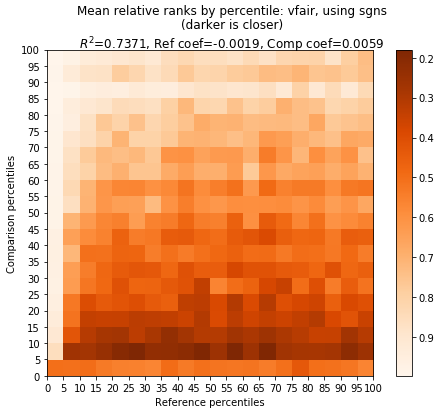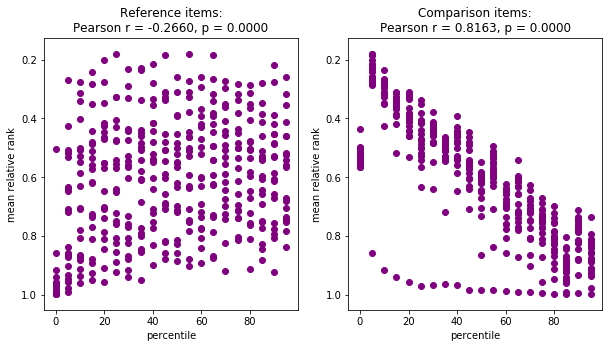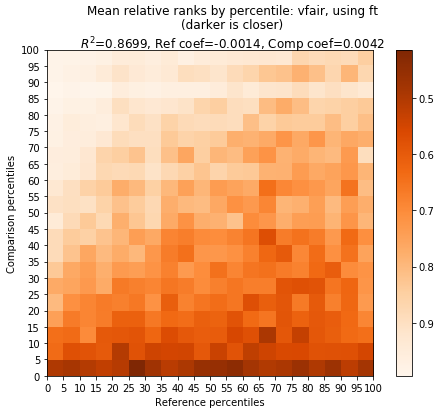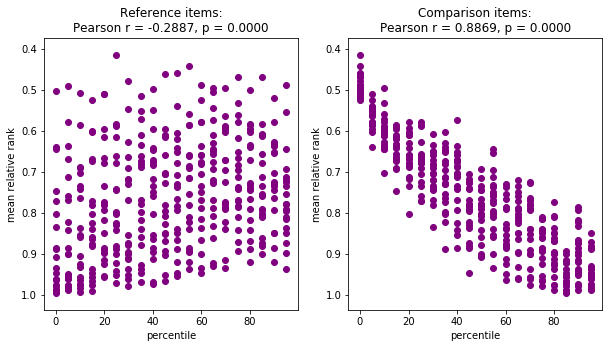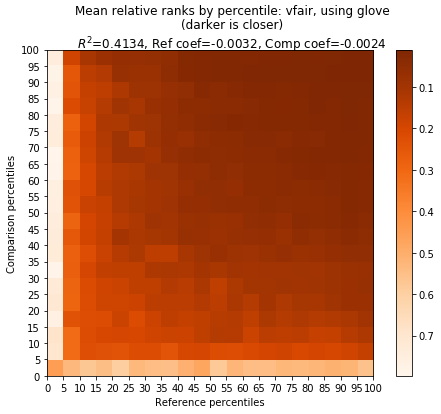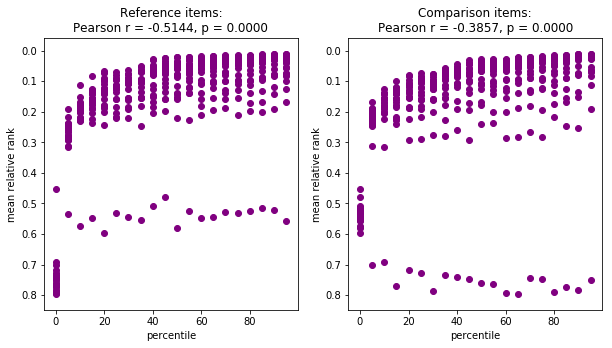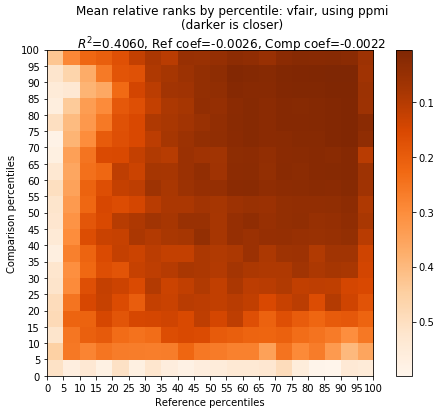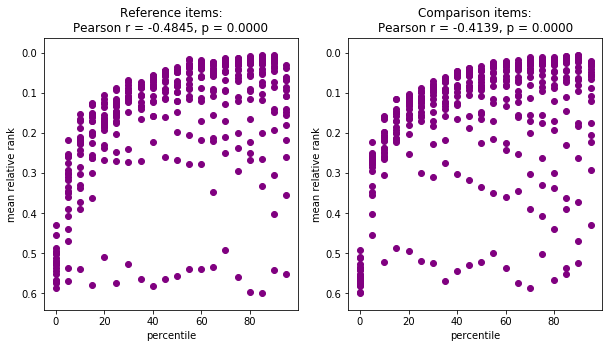There are three main things to notice in those examples. The first thing is that once again the sgns and ft models are similar to each other, and the glove and ppmi models are also similar to each other. The difference is that for glove and ppmi, the comparison terms have direct correlation between frequency and rank, unlike for sgns and ft.

The second thing is that reference terms have somewhat of a direct correlation between frequency and rank, across all 4 models.

The third thing is that the glove/ppmi pattern shows smaller differences between the reference and comparison results than does the sgns/ft patten. This suggests that in the glove and ppmi models, rank is in fact somewhat reciprocal.

We can examine reciprocity in more detail by looking at the magnitude of the difference in relative ranks when comparing $word_1$ and $word_2$ and vice versa. What do we expect? In geometric terms of the heatmaps above, what we are doing is comparing (by reflection across the diaganol of reference percentile = comparison percentile) the upper left diagonal with the lower right diagonal. The more alike the two halves are, the more reciprocal rank is. The two halves of sgns and ft are very different, so we expect a strong relation between frequency and reciprocity). On the other hand, the two halves of glove are very similar, so we expect litle relation between frequency and reciprocity. The two halves of ppmi are also similar, though not as similar as in the case of glove, so we might expect some kind of relation between reciprocity and frequency.

In fact, our expectations are mostly born out in the charts below. A dark diagonal from lower left to upper right compared to lighter other areas shows that items with similar frequencies (the ones near the diagonal) are more reciprocal than items with differing frequencies (the ones away from the diagonal). In other words, the reciprocity is limited to items of similar frequency. We see this pattern fairly clearly with sgns and ft, and we get pretty strong correlation (seen in the scatter plots) between frequency and reciprocity, as we predicted.

For glove, there is no pattern along the diagonal, and the correlation between frequency and reciprocity is low (~ 0.3), also as we expected. The one anomaly is that the lowest frequency items show less reciprocity than other items

The case of ppmi is a more puzzling, since there is somewhat of a dark diagonal, and there is a correlation between frequency and reciprocity is very similar to that for ft (~ 0.75 vs ~0.76). Visually, the heatmap and scatterplot for ppmi is different from that from sgns/ft, so this pattern deserves more attention, more than I can give here.

In :
samples = 100 #fewer, because this is slow
step = 5
Plotting.compare_recip_rank_comparisons(vfair_all,'vfair',samples=samples,step=step,full_range=False)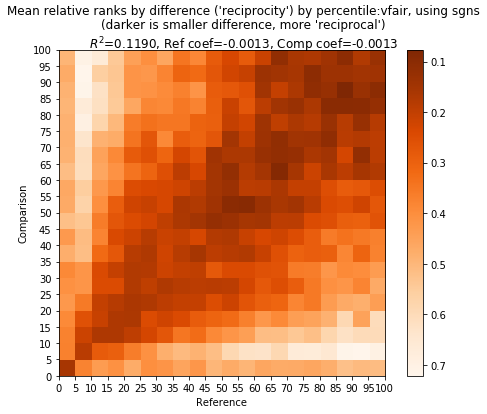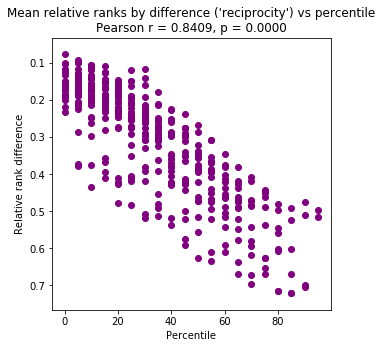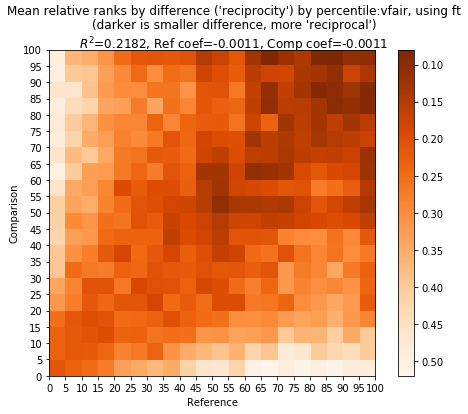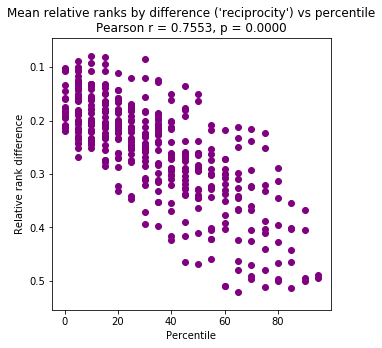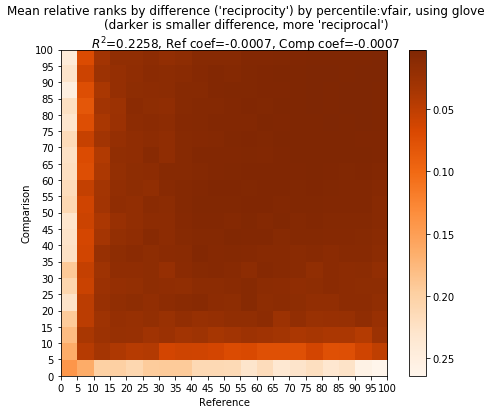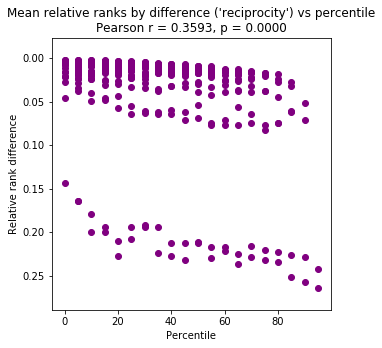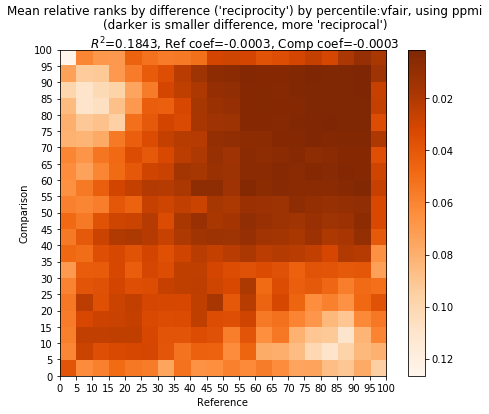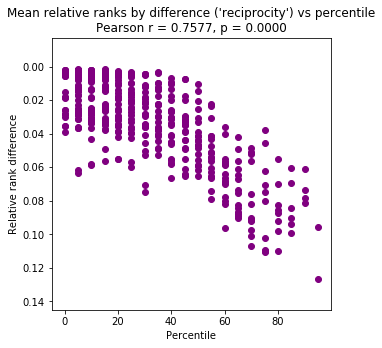Now let's see what happens with ranks in heartd.

In :
samples = 100 #fewer, because this is slow
step = 5
Plotting.compare_relrank_comparisons(heartd_all,'heartd', samples=samples, step=step, full_range=False)